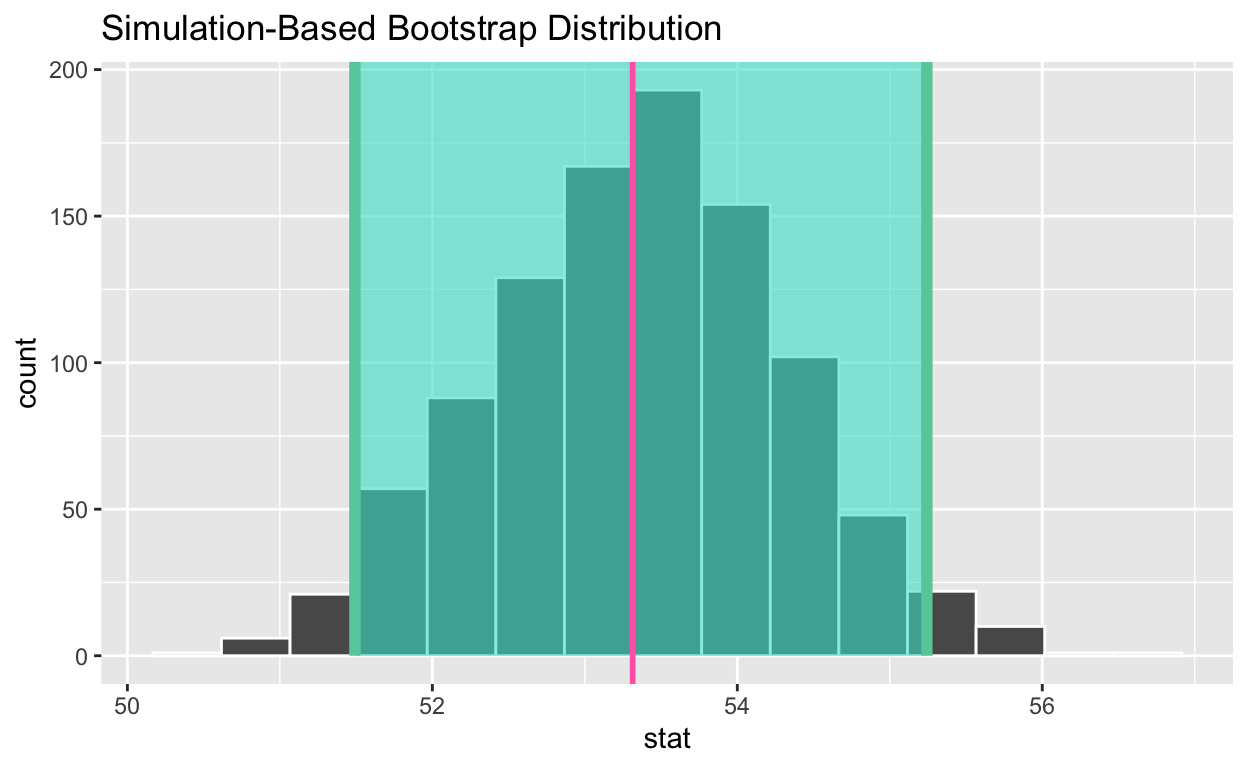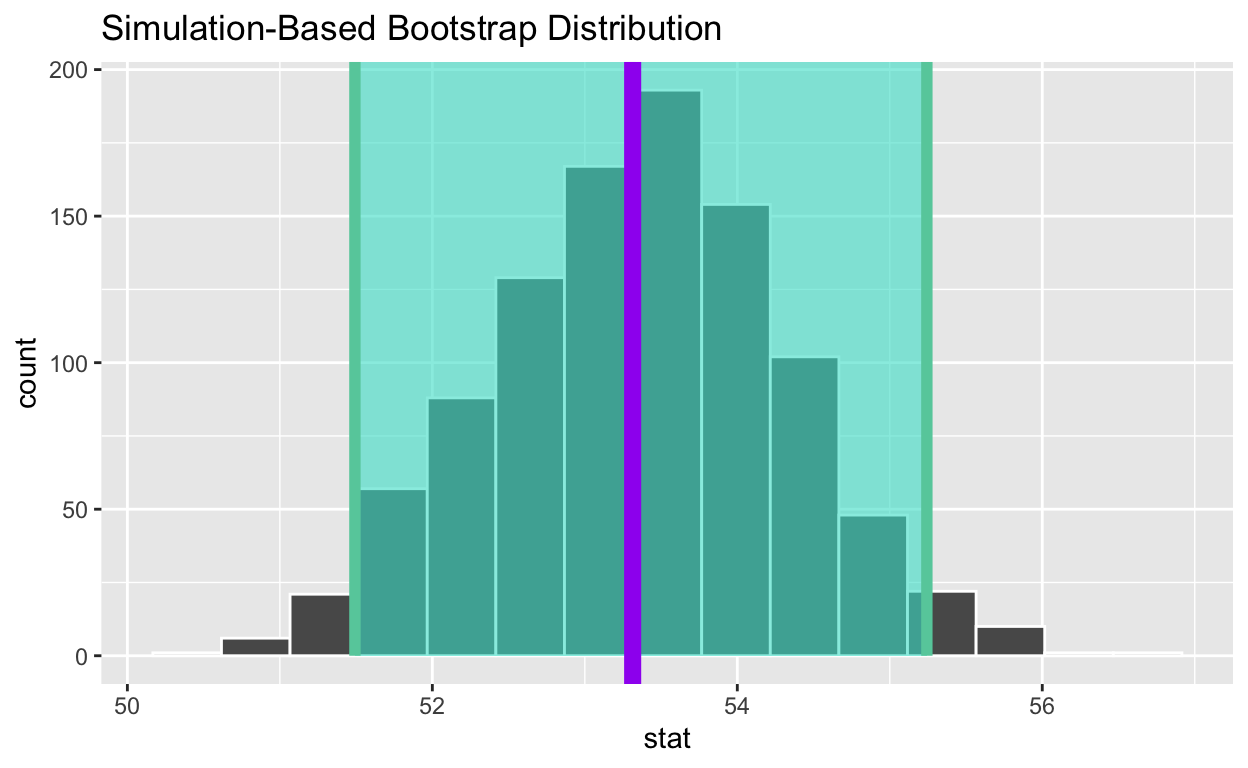# Bootstrapping

Based on Chapter 8 of ModernDive. Code for Quiz 12.

``````library(tidyverse)
library(moderndive)
library(infer)
library(fivethirtyeight)
``````

Replace all the instances of ???. These are answers on your moodle quiz.

Run all the individual code chunks to make sure the answers in this file correspond with your quiz answers

After you check all your code chunks run then you can knit it. It won’t knit until the ??? are replaced

Save a plot to be your preview plot

Look at the variable definitions in congress_age

What is the average age of members that have served in congress?

Set random seed generator to 123

Take a sample of 100 from the dataset congress_age and assign it to congress_age_100

``````set.seed(123)
congress_age_100 <- congress_age %>%
rep_sample_n(size=100)
``````

congress_age is the population and congress_age_100 is the sample.

18635 is number of observations in the the population and 100 is the number of observations in your sample.

Construct the confidence interval

1. Use specify to indicate the variable from congress_age_100 that you are interested in
``````congress_age_100  %>%
specify(response = age)
``````
``````Response: age (numeric)
# A tibble: 100 x 1
age
<dbl>
1  53.1
2  54.9
3  65.3
4  60.1
5  43.8
6  57.9
7  55.3
8  46
9  42.1
10  37
# … with 90 more rows``````
1. generate 1000 replicates of your sample of 100
``````congress_age_100  %>%
specify(response = age)  %>%
generate(reps = 1000, type= "bootstrap")
``````
``````Response: age (numeric)
# A tibble: 100,000 x 2
# Groups:   replicate [1,000]
replicate   age
<int> <dbl>
1         1  42.1
2         1  71.2
3         1  45.6
4         1  39.6
5         1  56.8
6         1  71.6
7         1  60.5
8         1  56.4
9         1  43.3
10         1  53.1
# … with 99,990 more rows``````

The output has 100,000 rows.

1. calculate the mean for each replicate

Assign to bootstrap_distribution_mean_age

Display bootstrap_distribution_mean_age

``````bootstrap_distribution_mean_age  <- congress_age_100  %>%
specify(response = age)  %>%
generate(reps = 1000, type = "bootstrap")  %>%
calculate(stat = "mean")
bootstrap_distribution_mean_age
``````
``````# A tibble: 1,000 x 2
replicate  stat
*     <int> <dbl>
1         1  53.6
2         2  53.2
3         3  52.8
4         4  51.5
5         5  53.0
6         6  54.2
7         7  52.0
8         8  52.8
9         9  53.8
10        10  52.4
# … with 990 more rows``````

The bootstrap_distribution_mean_age has 1,000 means.

1. visualize the bootstrap distribution

visualize(bootstrap_distribution_age)

Calculate the 95% confidence interval using the percentile method

Assign the output to congress_ci_percentile

Display congress_ci_percentile

``````congress_ci_percentile  <- bootstrap_distribution_mean_age %>%
get_confidence_interval(type = "percentile" , level =0.95)
congress_ci_percentile
``````
``````# A tibble: 1 x 2
lower_ci upper_ci
<dbl>    <dbl>
1     51.5     55.2``````

Calculate the observed point estimate of the mean and assign it to obs_mean_age

Display obs_mean_age,

``````obs_mean_age  <- congress_age %>%
specify(response = age) %>%
calculate(stat = "mean") %>%
pull()
obs_mean_age
``````
`` 53.31373``

 53.36 Shade the confidence interval

Add a line at the observed mean, obs_mean_age, to your visualization and color it “hotpink”

``````visualize(bootstrap_distribution_mean_age) +
geom_vline(xintercept = obs_mean_age, color = "hotpink", size = 1 )
``````Calculate the population mean to see if it is in the 95% confidence interval

Assign the output to pop_mean_age

Display pop_mean_age

``````pop_mean_age  <- congress_age %>%
summarize(pop_mean= mean(age))  %>% pull()
pop_mean_age
``````
`` 53.31373``

 53.31373 Add a line to the visualization at the, population mean, pop_mean_age, to the plot color it “purple”

``````visualize(bootstrap_distribution_mean_age) +